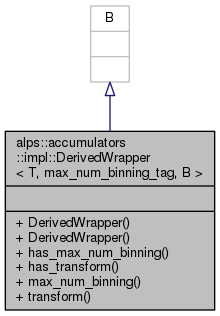ALPSCore reference
alps::accumulators::impl::DerivedWrapper< T, max_num_binning_tag, B > Class Template Reference

#include <max_num_binning.hpp>

Inheritance diagram for alps::accumulators::impl::DerivedWrapper< T, max_num_binning_tag, B >:Collaboration diagram for alps::accumulators::impl::DerivedWrapper< T, max_num_binning_tag, B >:## Public Member Functions

DerivedWrapper ()

DerivedWrapper (T const &arg)

bool has_max_num_binning () const

bool has_transform () const

max_num_binning_type< B >::type max_num_binning () const

void transform (boost::function< typename value_type< B >::type(typename value_type< B >::type)> op)

## Detailed Description

### template<typename T, typename B> class alps::accumulators::impl::DerivedWrapper< T, max_num_binning_tag, B >

Definition at line 437 of file max_num_binning.hpp.

## Constructor & Destructor Documentation

template<typename T , typename B >
 alps::accumulators::impl::DerivedWrapper< T, max_num_binning_tag, B >::DerivedWrapper ( )
inline

Definition at line 439 of file max_num_binning.hpp.

template<typename T , typename B >
 alps::accumulators::impl::DerivedWrapper< T, max_num_binning_tag, B >::DerivedWrapper ( T const & arg )
inline

Definition at line 440 of file max_num_binning.hpp.

## Member Function Documentation

template<typename T , typename B >
 bool alps::accumulators::impl::DerivedWrapper< T, max_num_binning_tag, B >::has_max_num_binning ( ) const
inline

Definition at line 442 of file max_num_binning.hpp.

template<typename T , typename B >
 bool alps::accumulators::impl::DerivedWrapper< T, max_num_binning_tag, B >::has_transform ( ) const
inline

Definition at line 443 of file max_num_binning.hpp.

template<typename T , typename B >
 max_num_binning_type::type alps::accumulators::impl::DerivedWrapper< T, max_num_binning_tag, B >::max_num_binning ( ) const
inline

Definition at line 445 of file max_num_binning.hpp.

template<typename T , typename B >
 void alps::accumulators::impl::DerivedWrapper< T, max_num_binning_tag, B >::transform ( boost::function< typename value_type< B >::type(typename value_type< B >::type)> op )
inline

Definition at line 446 of file max_num_binning.hpp.

The documentation for this class was generated from the following file: